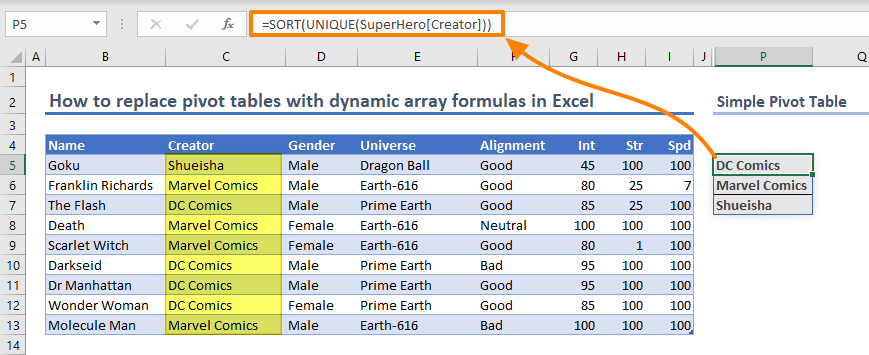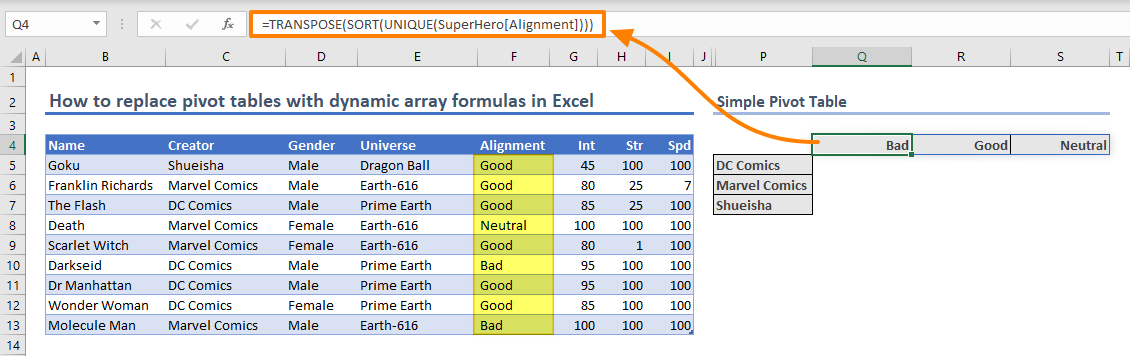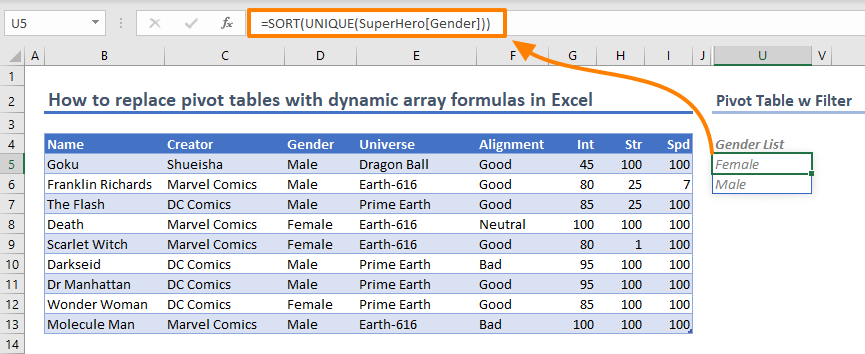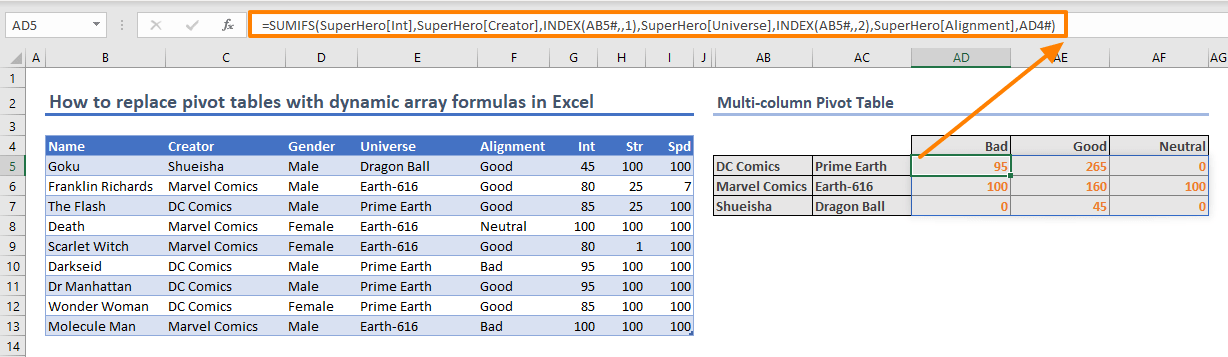Even though Pivot Tables are very useful in data management and analysis, they don't automatically update when you make changes. In this guide, we’re going to show you how to replace pivot tables with dynamic array formulas in Excel.

## Simple Pivot Table

The first step is to create a list for row and/or column titles. Then, you can use aggregation functions like SUMIFS or COUNTIFS.

Thanks to the dynamic array function UNIQUE, you can get a list of unique items from an array. Using the SORT or SORTBY functions these unique items can be sorted. The following formula lists unique items in a column in ascending order.

Rows: =SORT(UNIQUE(<column including row titles>))

By default, this formula returns values in a single column, because the data is inside a single column.If you need to use data with different column headers, you need to transpose the return array. Thanks to the dynamic arrays, the TRANSPOSE function does not require using the Ctrl + Shift + Enter combination anymore.

Wrap the row formula inside the TRANSPOSE function to spill the values into columns.

Columns: =TRANSPOSESORT(UNIQUE(<column including column titles>)))The final step is to use one of Excel’s aggregation functions which are,

Older counterparts of some of the formulas above like SUMIF, COUNTIF and AVEREAGEIF limit you to using a single condition.

Select the first (top-left) cell where the aggregated data is to be placed. The function must include the data column, as well as columns of row and column titles. The trick is selecting the entire title row or column when entering the data.

=SUMIFS(<column of data you want to aggregate>,<column including row titles>,<row headers>,<column including column titles>,<column headers>)If you check the formula, you can see that there are dash (#) characters next to the row and header titles: \$P\$5# and \$Q\$4#

The dash character is the spill range operator which indicates a spilled range. As a result, you can use a range without knowing where it ends. Now you have a dynamic pivot table!## Pivot Table with Filter

Next topic in how to replace pivot tables with dynamic array formulas article is adding filters. This feature can be mimicked by using formulas with certain limitations. Let’s see how you can add filter support in dynamic array pivot tables.

The first step is to generate the items to be used in filters. You can use the SORT and UNIQUE formulas, because items in the filter options must be unique and sorted.Select a cell you want to use as filter items and add a data validation list. You can open the dialog from Data > Data Validation command in the Ribbon.

In the Data Validation dialog, select List in the Allow box and enter the cell of the filter list formula with the spill operator. This action ensures to update your list each time the data is changed. Click OK to apply the settings.For adding filters, we need to add another criteria range-value pair. You need to add new functions into the formulas for the rows and column titles.

The FILTER function can return an array based on another array containing TRUE/FALSE values. Add a FILTER function into the SORT-UNIQUE combination to provide the filtered data to these functions.

Here is a basic syntax of filtered rows formula:

Rows: =SORT(UNIQUE(FILTER(<column including row titles>,<filter column>=<filter cell>)))

Although this formula is enough for listing the filtered rows, it doesn’t cover the scenario of an empty filter, or show all data without applying any filtering. You can handle both scenarios with a simple IF formula. The following formula can return all values (no filtering) if the filter cell is empty. The formula ensures this by sending a TRUE value when <filter cell> equals to an empty string (“”).

Rows: =SORT(UNIQUE(FILTER(<column including row titles>,IF(<filter cell>=””,TRUE,<filter column>=<filter cell>))))Please note that, we have named the filter cell, X5, as “Gender”.

Remember to add the FILTER function into column title formula as well.

For aggregation, you need to add the filter column and a simple condition for the filter cell as individual arguments. The condition makes the function search for not empty cells when the <filter cell> is empty.

=SUMIFS(<column of data you want to aggregate>,<column including row titles>,<row headers>,<column including column titles>,<column headers>,<filter column>,IF(<filter cell>=””,”<>”””””,<filter cell>))## Multi-column Pivot Table

Another useful feature of a Pivot Table is the multiple-field support for row and column titles. Excel can automatically group fields. You can drag and drop to add or remove fields. In this section of how to replace pivot tables with dynamic array formulas article, we will show you the multi-column support without filtering.

Let's assume that you want to add a field as the second-level row. You need use the UNIQUE function on both columns. The UNIQUE function evaluates every cell as a group in each row. This behavior returns a similar result to what you get in a Pivot Table.

The tricky part is giving multiple columns to the UNIQUE function. You can use the INDEX function which can return all rows of given columns. The INDEX function needs numbers and indexes to return data.

Multi-column rows: =SORT(UNIQUE(INDEX(<entire data>,<row numbers>,<column numbers>)))

Since all rows are required in the beginning, we need to number the rows, like 1 for the first row and 5 for the fifth. Instead of just putting these numbers, we can use the SEQUENCE function which returns an array of sequential numbers. With help of the ROWS function, the SEQUENCE formula can return high enough number to cover every row.

A static array can determine the columns. In our example, we used {2,4} to get the second and fourth columns.

Multi-column rows: =SORT(UNIQUE(INDEX(<entire data>,SEQUENCE(ROWS(<entire data>)),<array column numbers>)))Adding each column of the row titles as a separate criteria range-criteria value pairs will break the dynamic structure. Instead, you need a way to use the spilled data. The INDEX function can solve the problem in a similar way. Instead of static column references, using the INDEX function to return a row-title column by supplying column numbers.

=SUMIFS(<column of data you want to aggregate>,<column including row titles>,INDEX(<row titles>,,1),<column including row titles>,INDEX(<row titles>,,2),<column including column titles>,<column headers>)The empty argument of the INDEX function tells to return all rows. This doesn’t work for row titles due to the multiple number of columns.

## Multi-column Pivot Table w Filter

The final part of our how to replace pivot tables with dynamic array formulas in Excel article combining the previous steps. When the filter cell is empty, the formula should return a two-column array of TRUE instead of single TRUE value.

=SORT(UNIQUE(FILTER(INDEX(<entire data>,SEQUENCE(ROWS(<entire data>)),<array column numbers>),IF(<filter cell>="",{TRUE,TRUE},<filter column>=<filter cell>))))Since we have two columns, the array in our formula includes two TRUE values. Increase this number if you have more values.